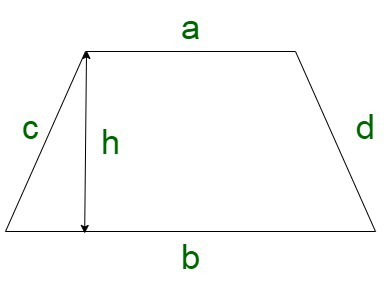# What is the formula to find the area of a Trapezium?

• Last Updated : 20 Nov, 2021

Trapezium which is also known as the trapezoid, is a closed quadrilateral, which contains a pair of parallel sides, whereas the other pair of sides are not parallel. The sides may or may not vary in length. The parallel sides of the trapezium are called the bases of the trapezium. The distance between the bases is known as the height of the trapezium. The height of the trapezium is also known as the altitude. The non-parallel sides of the trapezium are known as the legs.

The sum of interior angles of a trapezium is 360°. It has 4 sides and 4 vertices.Properties of trapezium

A trapezium is characterized by a set of following properties:

• The bases of a trapezium are parallel to each other.
• Both the diagonals are equal to each other.
• Sum of all interior angles is equivalent to 360o

### Area of trapezium

The area of any closed figure is the space enclosed within this object. In the case of trapezium, the area is given by,

Area = 1/2 × Sum of parallel sides × Distance between them.

Let us assume the a and b to be the parallel sides of the trapezium and h to be the distance between them. Let the area of trapezium be denoted by A.

We have,

A = 1/2 × (a + b) × d

On simplifying the equation, we have,

A =The area of the trapezium is denoted in square units.

### Perimeter of Trapezium

The perimeter of a trapezium is found by adding all the sides. Therefore, the perimeter of a trapezium formula is given as

The perimeter of Trapezium, P = a + b+ c + d units

Where,

“a, b, c, d” are the sides of the trapezium.

This formula used for the computation of the area of trapezium is known as the trapezium formula.

### Sample Questions

Question 1. Find the area of the trapezium in which parallel sides are 20 m and 25 m, its height is 20 m?

Solution:

Here.

To find the area of the trapezium

As we know that

Area of trapezium = 1/2 × (Sum of parallel side) × Height

Area of trapezium = 1/2 × (a + b) × Height

Where ‘a’ and ‘b’ are parallel sides

Given:

a = 20 m

b = 25 m

Height = 20 m

Now,

Area of trapezium = 1/2 × (20 + 25) × 20

Area of trapezium = 1/2 × (45) × 20

Area of trapezium = 450 m2

Therefore,

The area of trapezium is 450 m2.

Question 2. Find the sum of the parallel sides of the trapezium if its area is 1600 cm2 and height is 40 cm?

Solution:

Here we have to find the sum of the parallel sides of the trapezium.

As we know that

Area of trapezium = 1/2 × (Sum of parallel side) × Height

Given:

Area of trapezium = 1600 cm2

Height = 40 cm

Area of trapezium = 1/2 × (Sum of parallel side) × Height

1600 = 1/2 × (Sum of parallel side) × 40

Sum of parallel sides = (1600 × 2)/40

Sum of parallel sides = 80 cm

Therefore,

The sum of the parallel sides of the trapezium is 80 cm.

Question 3. Calculate the missing side of the trapezium if its perimeter is 110 m and the side are 20 m , 25 m and 30 m?

Solution:

Here,

We have to find the missing side of the trapezium.

As we know that

Perimeter of trapezium = Sum of all the sides

Assume the sides are a, b, c, and the missing side be d

Given :

a = 20 m

b = 25 m

c = 30 m

Perimeter of trapezium = a + b + c + d

110 = 20 + 25 + 30 + d

d = 110 – (20 + 25 + 30)

d = 110 – (75)

d = 35 m

Therefore,

The missing side of the trapezium is 35 m.

Question 4. Find the missing side and area of the isosceles trapezium if the perimeter of the trapezium is 250 cm and the sides are 40 cm, 60 cm, and 40 cm? Height of the trapezium is 50 cm.

Solution:

Here,

We have to find the missing side of the trapezium.

As we know that

Perimeter of trapezium = Sum of all the sides

Assume the sides are p, q, r and the missing side be ‘s’

Given :

p = 40 cm

q = 60 cm

r = 40 cm

Perimeter of trapezium = p + q + r + s

250 = 40 + 60 + 40 + s

s = 250 – (40 + 60 + 40)

s = 250 – (140)

s = 110 cm

Therefore,

The missing side of the trapezium is 110 cm.

Now,

Finding the area of the trapezium

As the perimeter is isosceles, so the nonequal side are parallel side

Parallel sides are

q = 60

s = 110

As we know that

Area of trapezium = 1/2 × (Sum of parallel side) × Height

Given :

Height = 50 cm

Area of trapezium = 1/2 × (60 + 110) × 50

Area of trapezium = 1/2 × (170) × 50

Area of trapezium = 4250 cm2.

My Personal Notes arrow_drop_up